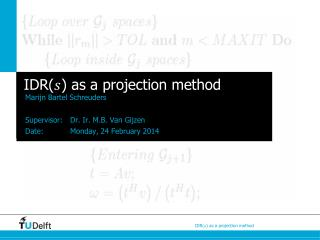# IDR( ) as a projection method - PowerPoint PPT PresentationDownload PresentationIDR( ) as a projection method

IDR( ) as a projection methodDownload Presentation## IDR( ) as a projection method

- - - - - - - - - - - - - - - - - - - - - - - - - - - E N D - - - - - - - - - - - - - - - - - - - - - - - - - - -
##### Presentation Transcript

1. IDR() as a projection method MarijnBartelSchreuders Supervisor: Dr. Ir. M.B. Van GijzenDate: Monday, 24 February 2014

2. Overview of this presentation • Iterative methods • Projection methods • Krylov subspace methods • Eigenvalue problems • Linear systems of equations • The IDR() method • General idea behind the IDR() method • Numerical examples • Ritz-IDR • Research Goals

3. Iterative methods • Consider a linear system (1) with and • Find an approximate solution to (1), with initial guess • Residual

4. Projection methods Subspaces • Define of dimension • ‘Subspace of candidate approximants’ or ‘Search subspace’ • Define of dimension • ‘Subspace of constraints’ or ‘Left subspace’

5. Projection methodsDefinition Find such that • Find • Let form an orthonormal basis for • Then How to find this vector?

6. Projection methods How to find • Let form an orthonormal basis for • Hence:

7. Projection methods General algorithm • How to choose the subspaces?

8. Krylov subspace methodsGeneral • Different methods for different choices of • Can be used for • eigenvalue problems • linear systems of equations

9. Krylov subspace methodsOverview

10. Krylov subspace methodsOverview

11. Krylov subspace methods Eigenvalue problems • Computing all eigenvalues can be costly • A is a full matrix • A is large • Idea: find smaller matrix for which it is easy to compute ‘Ritz values’ • Good approximations to some of the eigenvalues of A

12. Krylov subspace methodsOverview

13. Krylov subspace methodsOverview

14. Krylov subspace methods Symmetric matrices • Conjugate Gradient method (CG) • Optimality condition • Uses short recurrences • Minimises the residual

15. Krylov subspace methodsNonsymmetric matrices • GMRES-type methods • Long recurrences • Minimisation of the residual • Bi-CG – type methods • Short recurrences • No minimisation of the residual • Two matrix-vector operations per iteration • Are their any other possibilities?

16. Induced Dimension Reduction (s) • Residuals are forced to be in certainsubspaces • Compute residuals in each iteration

17. Induced Dimension Reduction (s)IDR theorem Theorem 1 (IDR theorem): Let and Let Let such that and do not share a subspace of Define: ) Then the following holds: (i) (ii) for some

18. Induced Dimension Reduction (s)Numerical experiments • Convection diffusion equation: • Discretise using finite differences on unit cube; Dirichlet boundary conditions • internal points equations • Stopping criterion:

19. Induced Dimension Reduction (s)Numerical experiments • This is an example of a slide

20. Induced Dimension Reduction (s)Numerical experiments • Matrix Market: matrix • Real, nonsymmetric, sparse matrix http://math.nist.gov/MatrixMarket/data/misc/hamm/add20.html

21. Induced Dimension Reduction (s)Numerical experiments • This is an example of a slide

22. Induced Dimension Reduction (s)Numerical experiments • This is an example of a slide

23. Induced Dimension Reduction (s)How to choose • Recall: ) • Minimisation of the residuals • Random? • …… ? How to choose ?

24. Induced Dimension Reduction (s)Ritz-IDR • Valeria Simoncini & Daniel Szyld • Ritz-IDR • Calculates Ritz values

25. Research goals • Research goals are twofold: • Make clear how we can see IDR() in the framework of projection methods • Use the IDR(s) algorithm for calculating the

26. IDR() as a projectionmethod MarijnBartelSchreuders Supervisor: Dr. Ir. M.B. Van GijzenDate: Monday, 24 February 2014

27. Research goals • Let • This is a polynomial in • To minimise, take derivative w.r.t.

28. Krylov subspace methods Eigenvalue problems Arnoldi Method Lanczos method & Bi-Lanczos method